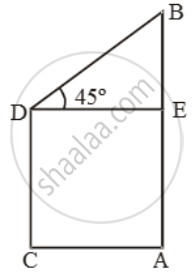# The heights of two poles are 80 m and 62.5 m. If the line joining their tops makes an angle of 45º with the horizontal, then find the distance between the pole - Mathematics

Sum

The heights of two poles are 80 m and 62.5 m. If the line joining their tops makes an angle of 45º with the horizontal, then find the distance between the pole

#### Solution

Let AB and CD be the poles such thatAB = 80 m and CD = 62.5 m.

Draw DE ⊥ AB. Then, ∠EDB = 45º

Now, BE = AB – AE = AB – CD = 17.5

\frac{DE}{BE}=\text{cot }45^\text{o}=1

⇒ DE = BE = 17.5 m

Concept: Heights and Distances
Is there an error in this question or solution?